# Statistics Assignment Help With Intra Class Correlation

Intra-class correlation means within class correlation. It is distinguishable from product moment correlation in as much as here both the variables measure the same characteristics. Sometimes specially in biological and agricultural study, it is of interest to know how the members of a family or group are correlated among themselves with respect to some one of their common characteristic. For example, we may require the require the correlation between the heights of brothers of a family or between yields of plots of an experimental block. In such cases both the variables measure the same characteristic, e.g., height and height or weight and weight. There is nothing to distinguish one from the other so that one may be treated as X-variable and the other as the Y-variable.Suppose we have A1, A2, …….,An families with K1, K2,……….,Kn members, each of which may be represented as

##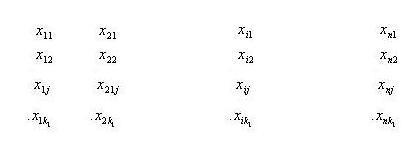and let xij(i=1,2,….n; j=1,2,……,ki) denote the measurement on the jth member in the ith family.

We shall have ki(ki-1) pairs for the ith family or group like (xij, xil),j≠1. There will beentries for all the n families or groups. The table is symmetrical about the principal diagonal. Such a table is called an intra-class correlation table and the correlation is called intra-class correlation.

In the bivariate table xi1 occurs (ki -1) times, xi2 occurs (ki -1) times,.xiki occurs (ki -1) times, i.e., from the ith family we have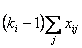and hence for all the n families we have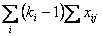as the marginal frequency, the table being symmetrical about principle diagonal.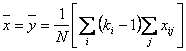Similarly,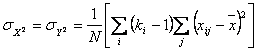Further

###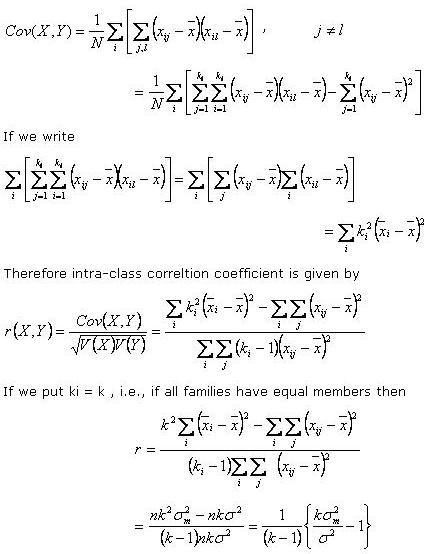Where σ2 denote the variance of X and σ2m the variance of means of families.